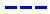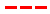Disneyland attraction wait times for November 11, 2023.

•Average Wait Time We PredictedAverage Wait Time We Saw
•Average Wait Time We PredictedAverage Wait Time We Saw
•Average Wait Time We PredictedAverage Wait Time We Saw
•Average Wait Time We PredictedAverage Wait Time We Saw
•Average Wait Time We PredictedAverage Wait Time We Saw
•Average Wait Time We PredictedAverage Wait Time We Saw
•Average Wait Time We PredictedAverage Wait Time We Saw
•Average Wait Time We PredictedAverage Wait Time We Saw
•Average Wait Time We PredictedAverage Wait Time We Saw
•Average Wait Time We PredictedAverage Wait Time We Saw
•Average Wait Time We PredictedAverage Wait Time We Saw
•Average Wait Time We PredictedAverage Wait Time We Saw
•Average Wait Time We PredictedAverage Wait Time We Saw
•Average Wait Time We PredictedAverage Wait Time We Saw
•Average Wait Time We PredictedAverage Wait Time We Saw
•Average Wait Time We PredictedAverage Wait Time We Saw
•Average Wait Time We PredictedAverage Wait Time We Saw
•Average Wait Time We PredictedAverage Wait Time We Saw
•Average Wait Time We PredictedAverage Wait Time We Saw
•Average Wait Time We PredictedAverage Wait Time We Saw
•Average Wait Time We PredictedAverage Wait Time We Saw
•Average Wait Time We PredictedAverage Wait Time We Saw
•Average Wait Time We PredictedAverage Wait Time We Saw
•Average Wait Time We PredictedAverage Wait Time We Saw
•Average Wait Time We PredictedAverage Wait Time We Saw
•Average Wait Time We PredictedAverage Wait Time We Saw
•Average Wait Time We PredictedAverage Wait Time We Saw
•Average Wait Time We PredictedAverage Wait Time We Saw
•Average Wait Time We PredictedAverage Wait Time We Saw
•Average Wait Time We PredictedAverage Wait Time We Saw
•Average Wait Time We PredictedAverage Wait Time We Saw
•Average Wait Time We PredictedAverage Wait Time We Saw
•Average Wait Time We PredictedAverage Wait Time We Saw
•Average Wait Time We PredictedAverage Wait Time We Saw
•Average Wait Time We PredictedAverage Wait Time We Saw
•Average Wait Time We PredictedAverage Wait Time We Saw